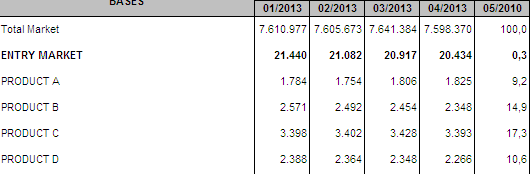# Qlik Design Blog

All about product and Qlik solutions: scripting, data modeling, visual design, extensions, best practices, etc.

Announcements
Support Case Portal has moved to Qlik Community! Read the FAQs to start exploring Support resources.Employee

## Accumulative Sums

We all use QlikCommunity when we need an answer for a QlikView question. We post a question and get an answer, usually in minutes, leveraging the expertise of 91,000+ QlikView users! QlikCommunity is the most-visited and active user community in the BI industry.

I also use QlikCommunity. And sometimes not to get an answer for a question but to think about new ways of doing things by reading the answers on the existing posts. So I have been curious about the answers on calculating the accumulative sums in QlikView and did a search on QlikCommunity. There are many answers provided as expected. Here is one way of achieving it by using rangesum() and aggr() functions.

It is a very common requirement to display the accumulative sum of a chart metric. The easiest way of doing this is to use the accumulate setting under the expressions tab of chart property.Figure 1. Accumulate option on chart property

With this setting, the values of the selected metric will be accumulated. But sometimes, the requirement is to have the first data point accumulated as well. For example, to show the accumulated sales for 12 months where the first month displayed should be the sum of the previous 12 months and the following months should accumulate on top of this value by adding one month at a time. One way of achieving this is to use the rangesum() and the aggr() functions together.

Let us first look at Rangesum(). It is a range function that returns the sum of a range of 1 to N arguments Together with the above() function, you can calculate the sum of the last 12 months;

rangesum( above( sum(Sales),0,12) )

This expression will return the sum of sales evaluated for each month as they appear on the 12 rows above the current row.

The next step is to combine RangeSum() with  the aggr() function to aggregate the sum of sales for the 12 months at the month level.

sum( aggr( rangesum( above( sum(Sales),0,12) ),Month))

Using the Aggr() function, it is possible to calculate the accumulated sum for any other dimensions in addition to  the time dimension. For example, the 12 months accumulated sum of sales by store can be calculated as;

sum( aggr( rangesum( above( sum(Sales),0,12) ),Month, Store))

Optionally you could also clear any selections on the month field as the calculation should reflect the accumulated sales for the previous 12 months, regardless of selections. This is achieved by using the set analysis “{\$<Month>}”.

sum( aggr( rangesum( above( sum( {\$<Month>}  Sales),0,12) ),Month))

One important thing to notice with this solution is the sort order inside the aggr() function. If the sort order is not correct, the aggregated sum of the 12 months would not be correct. QlikView always sorts the groupings (in this case the groups defined by the second parameter of the aggr() function) according to the load order. If the data is sorted by date during the load, QlikView will use this order and the aggr() function will show the correct totals for the desired time frame.

In summary, the accumulation option on the expression tab is the simplest way of achieving accumulations, but the use of rangesum() and aggr() functions are alternative ways of accumulating the data in more complex situations.

Tags (2)Employee

Nice one Elif!

One blog post for several dozens of replies in different places under different topics and subjects.

This issue is quite commonly asked, and thinking of rollings (months, years, periods, etc.), the value of your post becomes clear.

Thanks!

11,108 ViewsNot applicable

Excellent post Elif,

But got one question what if, I want to see the amounts of the 12 month sum, but not by rows but by columnsAs you can see, i have 6 products here, and those columns that each one shows, the sum of that month plus the 11 previous ones.

Any help in this case? any ideas?

Thank you very much.

11,108 ViewsPartner

In the expression used before:

rangesum( before( sum(Sales),0,12))

11,108 ViewsPartner

Very nice!

11,108 ViewsCreator II

Undoubtedly magnificent !

CB.

11,108 ViewsNot applicable

Hey guys,

I am given a task to calculate the frequency of calls across a territory. If the rep called a physician regarding the sale of the product 5 times, then frequency is 5 and HCP count is 1....I generated frequencies from 1 to 124 in my pivot table using a calculated dimension which is working fine. But my concern is :

My manager wants frequencies till 19 in order from 1..2..3..4...5..6.....19...

And from the frequency 21-124 as 20+.

I would be grateful if someone helps me with this.....Eager for the reply....

11,108 ViewsPartner

If(ColumnNo()=21,RangeCount(After(Count([Fact]),0,Max(total [Calculated Dimension])-20)),Count([Fact]))

8,475 ViewsPartner

In the expression above using the combination "ColumnNo() / after" if the value is calculated based on the values of the size of the graph as they appear in the next column, otherwise use "RowNo() / below" if the value is compared based on the values of the size of the graph as they appear in the next line.

8,475 ViewsNot applicable

I have used this calculated dimension to calculate the frequency

if(isnull(ENTITY_DISPLAY_NAME_VOD_C),aggr(if(count(v_ID_no_Parent)<>0,Count(v_id_no_Parent),[\$(vGrp)],PRIMARY_ACCOUNT_C),aggr(if(Count(v_ID_no_Parent)<>0,count(v_ID_no_Parent)),[\$(vGrp)],ENTITY_DISPLAY_NAME_VOD_C))

vgrp is for cyclic group ....v_id_no_Parent indicates calls ...rest is to calculate the right HCP count ....we are basically calculating frequencies of calls per territory..

Please help me with this...I need the solution asap...Can you people just modify the code according to the requirement...i tried...its not working...

8,475 ViewsPartner

You kindly send me a sample *.qvw file to make the changes. How well do you understand the problem ? Thanks you. AB

8,475 Views